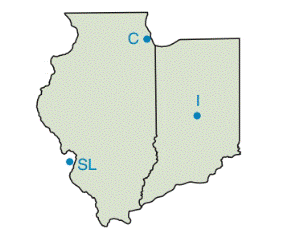Chapter 7.2, Problem 39E### Elementary Geometry for College St...

6th Edition
Daniel C. Alexander + 1 other
ISBN: 9781285195698

#### Solutions

Chapter
Section### Elementary Geometry for College St...

6th Edition
Daniel C. Alexander + 1 other
ISBN: 9781285195698
Textbook Problem
1 views

# A distributing company plans an Illinois location that would be the same distance from each of its principal delivery sites at Chicago, ST. Louis, and Indianapolis. Use a construction method to locate the approximate position of the distributing company.(Note: Trace the outline of the two states on your own paper.)To determine

To use:

The construction method to locate the approximate position of the distributing company.

Explanation

Definition:

Circumcenter:

The circumcenter of a triangle is the point in the plane equidistant from the three vertices of the triangle. Since a point equidistant from two points lies on the perpendicular bisector of the segment determined by the two points, the circumcenter is the point of concurrency of the three perpendicular bisectors of each side of the triangle.

Procedure used:

STEP 2: Construct the bisector of the line segment IL. Place the compasses on one end of the line segment. Set the compasses width to an approximately two thirds the line length. Without changing the compasses width, draw an arc above and below the line.

STEP 3: Again without changing the compasses width, place the compasses point on the other end of the line. Draw an arc above and below the line so that the arcs cross the first two.

STEP 4: Using a straightedge, draw a line between the points where the arcs intersect. This line is perpendicular to the first line and bisects it.

STEP 5: In the same manner, draw Construct the bisector of the line segment LC.

STEP 6: The point where these two perpendiculars intersect is the triangle's circumcenter.

Given:

A distributing company plans an Illinois location that would be the same distance from each of its principal delivery sites at Chicago, ST. Louis, and Indianapolis.

Clarification:

By the given diagram,

The point which is at same distance from the three cities, Chicago, ST. Louis, and Indianapolis is circumcenter of triangle formed by joining points of location of three cities.

So we construct the circumcenter of the triangle.

First we trace the point of location of three cities on the paper and join the three points as shown below.

### Still sussing out bartleby?

Check out a sample textbook solution.

See a sample solution

#### The Solution to Your Study Problems

Bartleby provides explanations to thousands of textbook problems written by our experts, many with advanced degrees!

Get Started

#### Find more solutions based on key concepts